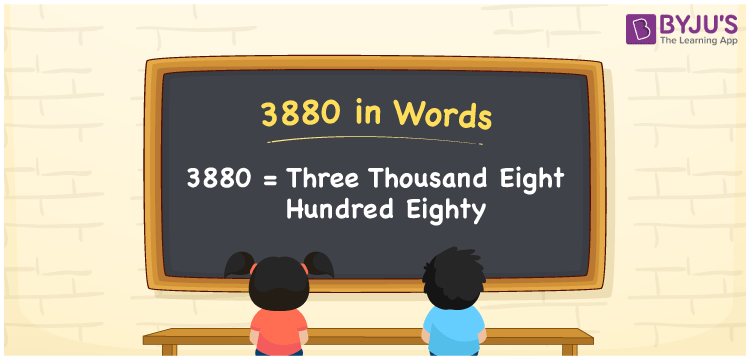# 3880 in words

3880 in words is written as Three Thousand Eight Hundred and Eighty. 3880 represents the count or value. The article on Counting Numbers can give you an idea about count or counting. The number 3880 is used in expressions that relate to money, distance, length, year and others. Let us consider an example for 3880. ”A remote village has Three Thousand Eight Hundred and Eighty houses”.

 3880 in words Three Thousand Eight Hundred and Eighty Three Thousand Eight Hundred and Eighty in Numbers 3880

## 3880 in English Words## How to Write 3880 in Words?

We can convert 3880 to words using a place value chart. The number 3880 has 4 digits, so let’s make a chart that shows the place value up to 4 digits.

 Thousands Hundreds Tens Ones 3 8 8 0

Thus, we can write the expanded form as:

3 × Thousand + 8 × Hundred + 8 × Ten + 0 × One

= 3 × 1000 + 8 × 100 + 8 × 10 + 0 × 1

= 3880

= Three Thousand Eight Hundred and Eighty.

3880 is the natural number that is succeeded by 3879 and preceded by 3881.

3880 in words – Three Thousand Eight Hundred and Eighty.

Is 3880 an odd number? – No.

Is 3880 an even number? – Yes.

Is 3880 a perfect square number? – No.

Is 3880 a perfect cube number? – No.

Is 3880 a prime number? – No.

Is 3880 a composite number? – Yes.

## Solved Example

1. Write the number 3880 in expanded form

Solution: 3 × 1000 + 8 × 100 + 8 × 10 + 0 × 1

We can write 3880 = 3000 + 800 + 80 + 0

= 3 × 1000 + 8 × 100 + 8 × 10 + 0 × 1

## Frequently Asked Questions on 3880 in words

Q1

### How to write 3880 in words?

3880 in words is written as Three Thousand Eight Hundred and Eighty.
Q2

### Is 3880 a perfect square number?

No. 3880 is not a perfect square number.
Q3

### Is 3880 a prime number?

No. 3880 is not a prime number.